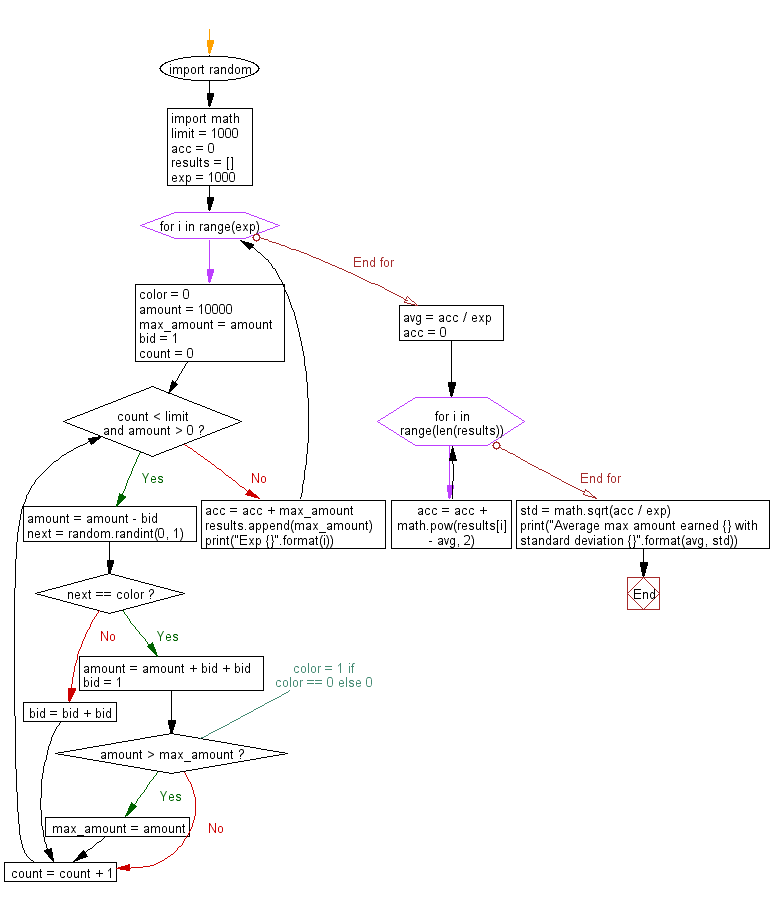﻿ Python Math: Casino simulation - w3resource# Python Math: Casino simulation

## Python Math: Exercise-70 with Solution

Write a Python program for casino simulation.

Sample Solution:-

Python Code:

``````#https://gist.github.com/ivanGzz/243a453ba6499a49027c19cac60471f6
import random
import math

limit = 1000
acc = 0
results = []
exp = 1000

for i in range(exp):
color = 0
amount = 10000
max_amount = amount
bid = 1
count = 0
while count < limit and amount > 0 :
amount = amount - bid
next = random.randint(0, 1)
if next == color :
amount = amount + bid + bid
bid = 1
# color = 1 if color == 0 else 0
if amount > max_amount:
max_amount = amount
else :
bid = bid + bid
count = count + 1
acc = acc + max_amount
results.append(max_amount)
print("Exp {}".format(i))

avg = acc / exp
acc = 0
for i in range(len(results)):
acc = acc + math.pow(results[i] - avg, 2)
std = math.sqrt(acc / exp)

print("Average max amount earned {} with standard deviation {}".format(avg, std))
```
```

Sample Output:

```Exp 0
Exp 1
Exp 2
Exp 3
Exp 4
Exp 5
----
Exp 998
Exp 999
Average max amount earned 10489.705 with standard deviation 56.22367806360589
```

Flowchart:## Visualize Python code execution:

The following tool visualize what the computer is doing step-by-step as it executes the said program:

Python Code Editor:

Have another way to solve this solution? Contribute your code (and comments) through Disqus.

What is the difficulty level of this exercise?

Test your Programming skills with w3resource's quiz.

﻿

## Python: Tips of the Day

Performs left-to-right function composition:

Example:

```from functools import reduce

def tips_compose_right(*fns):
return reduce(lambda f, g: lambda *args: g(f(*args)), fns)
add = lambda x, y: x + y
square = lambda x: x * x
```49